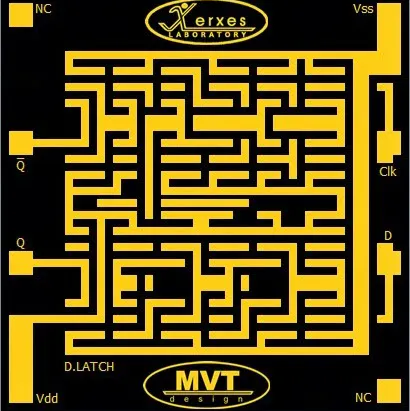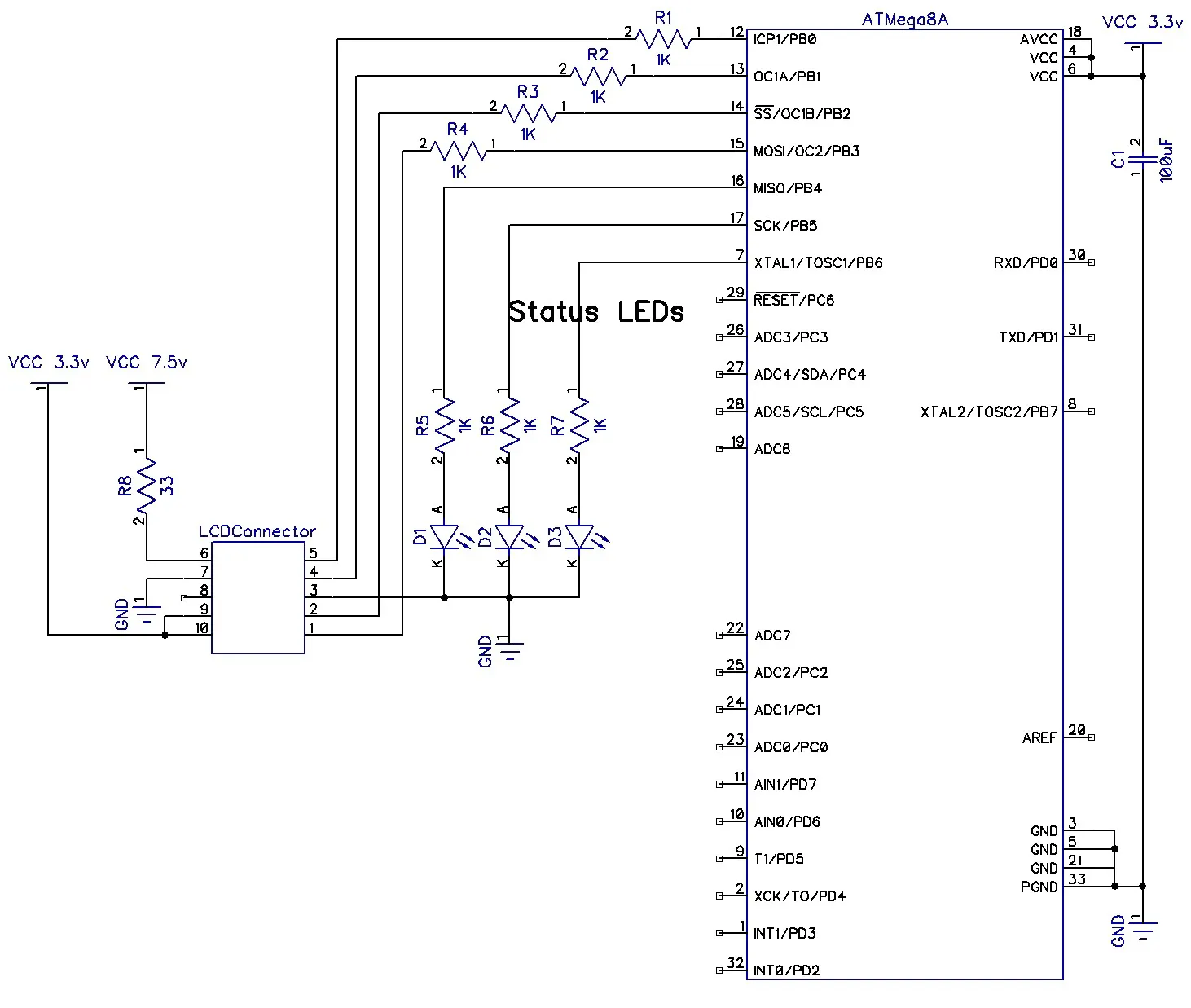# How to Drive Nokia Tiny LCDs

Here's a simple circuit and code which is compatible with PCF8833 driver for old Nokia tiny LCDs such as 1208, 1600, 2126, 2310, 6100, 6125, N71.

IntermediateFull instructions provided1 hour1,043

## Things used in this project

### Hardware components

 Microchip ATMega 8A
×1

## Schematics## Code

### Code:

C/C++
```/*******************************************************
This program was created by the

Chip type               : ATmega8A
AVR Core Clock frequency: 8.000000 MHz (Internal)
*******************************************************/

#include <mega8.h>

register unsigned char		ucI,rucJ,rucK,i,j;

void vCMD();
void vData();
void vSend();
void vClk();
void vDelay();

// 1mS Timer 0 overflow interrupt service routine
interrupt [TIM0_OVF] void timer0_ovf_isr(void)
{
rucJ++;
TIFR = 0x01;
}

void main(void)
{
//Microcontroller init
PORTB = 0x0A;
DDRB = 0x3F;

OSCCAL = 0x99;

//Low level external interrupts - sleep enable
MCUCR = 0x8A;

//Timer 0
TCNT0 = 0;
TCCR0 = 0x03;
TIFR = 0x01;
TIMSK = 0x01;

#asm("sei");

//PORTB.PORTB0		//RST#
//PORTB.PORTB1		//CS#
//PORTB.PORTB2		//SI
//PORTB.PORTB3		//SCK

PORTB.PORTB0 = 0;		//RST#
rucK = 250;
//1 second delay
vDelay();
vDelay();
vDelay();
vDelay();

PORTB.PORTB4 = 1; //Status LED 1 On
vDelay();

//LCD Reset
rucK = 1;
PORTB.PORTB0 = 1;		//RST#
vDelay();
PORTB.PORTB0 = 0;		//RST#
vDelay();
PORTB.PORTB0 = 1;		//RST#

//LCD Init
ucI = 0x11;
vCMD();

ucI = 0x20;
vCMD();

ucI = 0x3A;
vCMD();
ucI = 0x05;
vData();

ucI = 0x36;
vCMD();
ucI = 0xC8;
vData();

ucI = 0x25;
vCMD();
ucI = 0x30;
vData();

ucI = 0x29;
vCMD();

PORTB.PORTB5 = 1;
rucK = 250;
vDelay();

//98*70
ucI = 0x2A;
vCMD();
ucI = 0;
vData();
ucI = 97;
vData();

ucI = 0x2B;
vCMD();
ucI = 0;
vData();
ucI = 69;
vData();

//0xFFFF White
//0x0000 Black

while(1)
{
ucI = 0x2c; // Screen data command
vCMD();

//First line
//Border white
for(j = 0; j < 98; j++)
{
//1st byte: BBBBB GGG
//2nd byte: GGG RRRRR
ucI = 0xFF;
vData();
ucI = 0xFF;
vData();
}

//Second line
ucI = 0xFF;
vData();
ucI = 0xFF;
vData();

//Border Black
for(j = 0; j < 96; j++)
{
//1st byte: BBBBB GGG
//2nd byte: GGG RRRRR
ucI = 0x00;
vData();
ucI = 0x00;
vData();
}

ucI = 0xFF;
vData();
ucI = 0xFF;
vData();

//Blue screen
for(i = 0; i < 66; i++)
{
//Border
ucI = 0xFF;
vData();
ucI = 0xFF;
vData();
ucI = 0x00;
vData();
ucI = 0x00;
vData();

for(j = 0; j < 94; j++)
{
//1st byte: BBBBB GGG
//2nd byte: GGG RRRRR
ucI = 0xF8;
vData();
ucI = 0x00;
vData();
}
ucI = 0x00;
vData();
ucI = 0x00;
vData();
ucI = 0xFF;
vData();
ucI = 0xFF;
vData();
}

//Border
ucI = 0xFF;
vData();
ucI = 0xFF;
vData();

for(j = 0; j < 96; j++)
{
//1st byte: BBBBB GGG
//2nd byte: GGG RRRRR
ucI = 0x00;
vData();
ucI = 0x00;
vData();
}
ucI = 0xFF;
vData();
ucI = 0xFF;
vData();

//White border
for(j = 0; j < 98; j++)
{
//1st byte: BBBBB GGG
//2nd byte: GGG RRRRR
ucI = 0xFF;
vData();
ucI = 0xFF;
vData();
}

vDelay(); //250 mS Delay

//Green screen
ucI = 0x2c; // Screen data command
vCMD();

for(i = 0; i < 70; i++)
{
for(j = 0; j < 98; j++)
{
ucI = 0x07;
vData();
ucI = 0xE0;
vData();
}
}

vDelay(); // 250mS Delay

//Red Screen
ucI = 0x2c; // Screen data command
vCMD();

for(i = 0; i < 70; i++)
{
for(j = 0; j < 98; j++)
{
ucI = 0x00;
vData();
ucI = 0x1F;
vData();
}
}

vDelay(); //250mS
};
}

void vCMD()
{
PORTB.PORTB1 = 0;		//CS#

#asm("NOP");
#asm("NOP");
#asm("NOP");
#asm("NOP");

PORTB.PORTB2 = 0;		//SI#

#asm("NOP");
#asm("NOP");
#asm("NOP");
#asm("NOP");

vSend();
}

void vData()
{
PORTB.PORTB1 = 0;		//CS#

#asm("NOP");
#asm("NOP");
#asm("NOP");
#asm("NOP");

PORTB.PORTB2 = 1;		//SI#

#asm("NOP");
#asm("NOP");
#asm("NOP");
#asm("NOP");

vSend();
}

void vSend()
{
vClk();

if(ucI & 0x80)
PORTB.PORTB2 = 1;		//SI#
else
PORTB.PORTB2 = 0;		//SI#

vClk();

if(ucI & 0x40)
PORTB.PORTB2 = 1;		//SI#
else
PORTB.PORTB2 = 0;		//SI#

vClk();

if(ucI & 0x20)
PORTB.PORTB2 = 1;		//SI#
else
PORTB.PORTB2 = 0;		//SI#

vClk();

if(ucI & 0x10)
PORTB.PORTB2 = 1;		//SI#
else
PORTB.PORTB2 = 0;		//SI#

vClk();

if(ucI & 0x08)
PORTB.PORTB2 = 1;		//SI#
else
PORTB.PORTB2 = 0;		//SI#

vClk();

if(ucI & 0x04)
PORTB.PORTB2 = 1;		//SI#
else
PORTB.PORTB2 = 0;		//SI#

vClk();

if(ucI & 0x02)
PORTB.PORTB2 = 1;		//SI#
else
PORTB.PORTB2 = 0;		//SI#

vClk();

if(ucI & 0x01)
PORTB.PORTB2 = 1;		//SI#
else
PORTB.PORTB2 = 0;		//SI#

vClk();

PORTB.PORTB1 = 1;		//CS#
}

void vClk()
{
PORTB.PORTB3 = 0;		//SCK#

#asm("NOP");
#asm("NOP");
#asm("NOP");
#asm("NOP");
PORTB.PORTB3 = 1;		//SCK#

#asm("NOP");
#asm("NOP");
#asm("NOP");
#asm("NOP");
}

void vDelay()
{
rucJ = 0;

while(rucJ < rucK) //100 mS delay
{
#asm("sleep");
};
}
```

## Credits

### M. Mahdi K. Kanan

30 projects • 14 followers
M. Mahdi K. Kanan is the manager of WiCardTech engineering group.